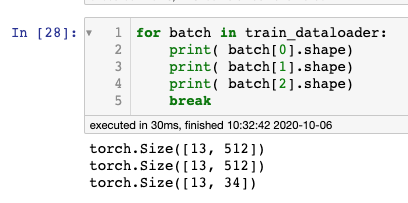# Batch size keeps on changin, throwing `Pytorch Value Error Expected: input batch size does not match target batch size`

I am working on a multilabel text classification task with Bert.

The following is the code for generating an iterable Dataset.

``````from torch.utils.data import TensorDataset, DataLoader, RandomSampler, SequentialSampler

train_set = TensorDataset(X_train_id,X_train_attention, y_train)
test_set = TensorDataset(X_test_id,X_test_attention,y_test)

train_set,
sampler = RandomSampler(train_set),
drop_last=True,
batch_size=13
)

test_set,
sampler = SequentialSampler(test_set),
drop_last=True,
batch_size=13
)
``````

The following are the the dimensions of the training set:

In[]

``````print(X_train_id.shape)
print(X_train_attention.shape)
print(y_train.shape)
``````

Out[]

``````torch.Size([262754, 512])
torch.Size([262754, 512])
torch.Size([262754, 34])
``````

There should be 262754 rows each with 512 columns. The output should predict the values from 34 possible labels. I am breaking them down into batches of 13.

Training code

``````optimizer = AdamW(model.parameters(), lr=2e-5)
# Training
def train(model):
model.train()
train_loss = 0
b_input_ids = batch.to(device)
b_labels = batch.to(device)
loss, logits = model(b_input_ids,
token_type_ids=None,
labels=b_labels)
loss.backward()
optimizer.step()
train_loss += loss.item()
return train_loss

# Testing
def test(model):
model.eval()
val_loss = 0
b_input_ids = batch.to(device)
b_labels = batch.to(device)
(loss, logits) = model(b_input_ids,
token_type_ids=None,
labels=b_labels)
val_loss += loss.item()
return val_loss

max_epoch = 1
train_loss_ = []
test_loss_ = []

for epoch in range(max_epoch):
train_ = train(model)
test_ = test(model)
train_loss_.append(train_)
test_loss_.append(test_)

``````

Out[]

``````Expected input batch_size (13) to match target batch_size (442).
``````

This is the description of my model:

``````from transformers import BertForSequenceClassification, AdamW, BertConfig

model = BertForSequenceClassification.from_pretrained(
num_labels = 34,
output_attentions = False,
output_hidden_states = False,
)
``````

I have clearly stated that I want the batch size to be 13. However, during the training process pytorch is throwing a Runtime Error

Where is the number 442 even coming from? I have clearly stated that I want each batch to have a size of 13 rows.

I have already confirmed that each batch has input_id with dimensions [13,512], attention tensor with dimensions [13,512], and labels with dimensions [13,34].

I have tried caving in and using a batch size of 442 when initializing the DataLoader, but after a single batch iteration, it throws another `Pytorch Value Error Expected: input batch size does not match target batch size`, this time showing:

``````ValueError: Expected input batch_size (442) to match target batch_size (15028).
``````

Why does the batch size keep on changing? Where is the number 15028 even coming from?

The following are some of the answers I have looked through, but had no luck on applying to my source code because none of them talk about batch sizes changing:

Thanks in advance. Your support is truly appreciatedI think it’s the flattened target tensor: `[13*43=442]`.
Based on the docs for `BertForSequenceClassification`, the targets are expected as `[batch_size]` so they are most likely internally flattened.

I’m not familiar with the implementation, but since it’s a classification use case I guess the targets have the same (or similar) requirements as the targets for `nn.CrossEntropyLoss`.
I.e. they are expected in the shape `[batch_size]` as `LongTensor`s containing the class indices in the range `[0, nb_classes-1]` for a multi-class classification use case.

Thanks for the feedback.

However, I have confirmed that during each iteration during the training, my dimensions are:

input_ids: [13,512]
y_labels: [13,34]

where 34 is the number of possible labels (as one hot vectors).
Ex:
[0,0,0,1,1,0,0,… 0,1]

Doesn’t this meet the requirement for calculating Cross EntropyLoss ?No, since class labels are expected.
Use `target = torch.argmax(target, dim=1)` to create the expected target tensor.

1 Like